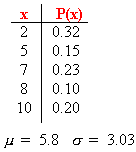### CFA Practice Question

There are 985 practice questions for this topic.

### CFA Practice Question

For the probability distribution shown below, the mean and standard deviation has been computed. The expected value of random variable x is ______.A. 0.15
B. 3.03
C. 5.8